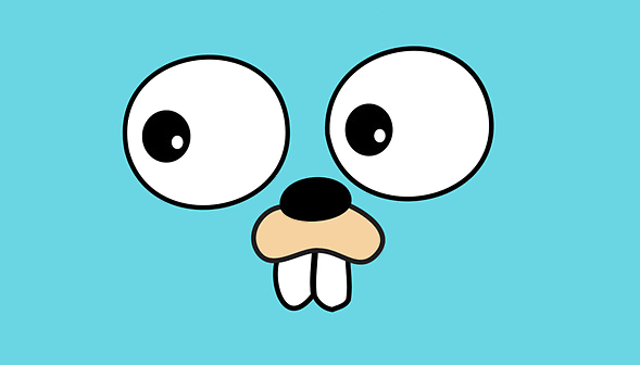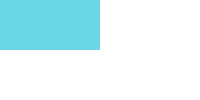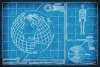# Create a new image

yourbasic.org/golangUse the `image`, `image/color`, and `image/png` packages to create a PNG image.

``````width := 200
height := 100

upLeft := image.Point{0, 0}
lowRight := image.Point{width, height}

img := image.NewRGBA(image.Rectangle{upLeft, lowRight})

// Colors are defined by Red, Green, Blue, Alpha uint8 values.
cyan := color.RGBA{100, 200, 200, 0xff}

// Set color for each pixel.
for x := 0; x < width; x++ {
for y := 0; y < height; y++ {
switch {
case x < width/2 && y < height/2: // upper left quadrant
img.Set(x, y, cyan)
case x >= width/2 && y >= height/2: // lower right quadrant
img.Set(x, y, color.White)
default:
// Use zero value.
}
}
}

// Encode as PNG.
f, _ := os.Create("image.png")
png.Encode(f, img)``````

Output (image.png):

``````

Note: The upper right and lower left quadrants of the image are transparent (the alpha value is 0) and will be the same color as the background.

## Go image support

The `image` package implements a basic 2-D image library without painting or drawing functionality. The article The Go image package has a nice introduction to images, color models, and image formats in Go.

Additionally, the `image/draw` package provides image composition functions that can be used to perform a number of common image manipulation tasks. The article The Go image/draw package has plenty of examples.

### More code examplesGo blueprints: code for com­mon tasks is a collection of handy code examples.